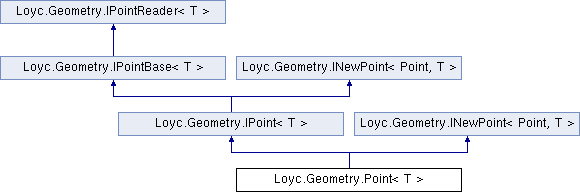Enhanced C# Language of your choice: library documentation

# Documentation moved to ecsharp.net

GitHub doesn't support HTTP redirects, so you'll be redirected in 3 seconds.

Loyc.Geometry.Point< T > Struct Template Reference

A 2D point (X-Y pair) structure. More...

Source file:
Inheritance diagram for Loyc.Geometry.Point< T >:## Remarks

A 2D point (X-Y pair) structure.

Although this structure contains operators such as + and -, their performance is suboptimal due to limitations of C#. The PointMath class of Loyc.Utilities.dll contains extension methods such as Add() and Sub() that perform faster on common coordindate types such as int and double.

Template Parameters
 T Coordinate type.
PointMath, Vector{T}
Type Constraints
 T : IConvertible T : IEquatable

_x

_y

## Public static fields

static IMath< T > m = Maths<T>.Math

static readonly Point< T > Zero = new Point<T>()

static readonly Point< T > Inf = new Point<T>(m.PositiveInfinity, m.PositiveInfinity)

## Properties

X` [get, set]`

Y` [get, set]`Properties inherited from Loyc.Geometry.IPointBase< T >
new T X` [get, set]`
Horizontal coordinate of a point or vector. More...

new T Y` [get, set]`
Vertical coordinate of a point or vector. More...Properties inherited from Loyc.Geometry.IPointReader< T >
X` [get]`

Y` [get]`

## Public Member Functions

Point (T x, T y)

override bool Equals (object other)

override int GetHashCode ()

override string ToString ()

Point< T > New (T x, T y)

IPoint< T > INewPoint< IPoint
< T >, T >.
New (T x, T y)

bool Equals (Point< T > other)

LineSegment< T > To (Point< T > other)

## Static Public Member Functions

static operator Vector< T > (Point< T > p)

static operator Point< int > (Point< T > p)

static operator Point< long > (Point< T > p)

static operator Point< float > (Point< T > p)

static implicit operator Point< double > (Point< T > p)

static operator System.Drawing.Point (Point< T > p)

static operator System.Drawing.PointF (Point< T > p)

static operator System.Windows.Point (Point< T > p)

static Point< T > operator+ (Point< T > a, Vector< T > b)

static Point< T > operator+ (Vector< T > a, Point< T > b)

static Point< T > operator- (Point< T > a, Vector< T > b)

static Vector< T > operator- (Point< T > a, Point< T > b)

static Point< T > operator* (Point< T > p, T factor)

static Point< T > operator/ (Point< T > p, T factor)

static Point< T > operator<< (Point< T > p, int amt)

static Point< T > operator>> (Point< T > p, int amt)

static bool operator== (Point< T > a, Point< T > b)

static bool operator!= (Point< T > a, Point< T > b)